# Math > Year 2

## Interpreting Pictograms

What is a pictogram?

A pictogram is a graph that uses pictures to represent data.

A pictogram is also known as a pictograph or picto.

Here is an example of a pictogram.
In the given pictogram, data related to the favourite snacks of different children is shown.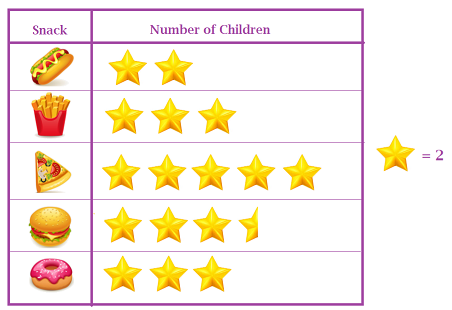Here, one star means two children.

A half star means half of 2 i.e. 1.

Number of children who like Hotdog =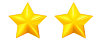= 2 + 2 = 4

Number of children who like Fries =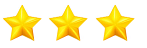= 2 + 2 + 2 = 6

Number of children who like Pizza =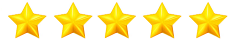= 2 + 2 +2 +2 + 2 = 10

Number of children who like Burger =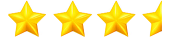= 2 + 2 + 2 + 1 = 7

Number of children who like Doughnut  == 2 + 2 + 2 = 6

Which is the least favourite snack?  - Hotdog

//